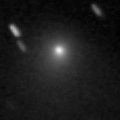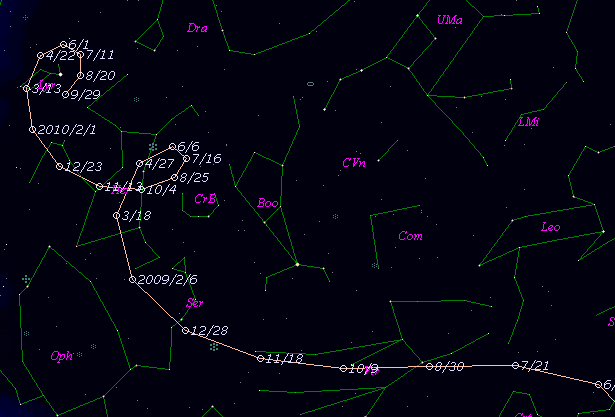# \$B%^%/%N!<%HWB@1(B

C/2006 Q1 ( McNaught )###\$B%W%m%U%#!<%k(B

 \$BH/8+F|(B 2006\$BG/(B8\$B7n(B20\$BF|(B \$BH/8+8wEY(B 17.8\$BEy(B \$BH/8+ R. H. McNaught (Siding Spring Survey)

###\$B###\$B50F;MWAG(B

```The following orbital elements are taken from MPC 72849:

Epoch 2008 June 23.0 TT = JDT 2454640.5
T 2008 July 3.8554 TT                                   MPC
q   2.763665             (2000.0)            P               Q
z  +0.000068       Peri.  344.3796      -0.9539012      -0.0880220
+/-0.000000       Node   199.5463      -0.0840291      -0.8394568
e   0.999812       Incl.   59.0471      -0.2881174      +0.5362503
From 2552 observations 2006 Aug. 20-2010 Oct. 17, mean residual 0".5.
```

###\$B@1?^(B###\$B8wEYJQ2=(B

```        m1 = 4.8 + 5 log\$B&\$(B + 10.0 log r  [ ,0]  (              \$B!A(B2008\$BG/(B 7\$B7n(B 3\$BF|(B)
m1 = 4.3 + 5 log\$B&\$(B + 11.0 log r  [0, ]  (2008\$BG/(B 7\$B7n(B 3\$BF|!A(B              )
```##### \$B50F;MWAG\$O(BMPC\$B\$N(BEphemerides and Orbital Elements\$B\$K7G:\\$5\$l\$?\$b\$N\$G\$9!#(B \$B@1?^\$O(B StellaNavigator Ver.2.0 for Windows (\$B%"%9%H%m%"!<%D(B \$BJTCx(B / \$B%"%9%-!<=PHG6I4)(B) \$B\$G:n@.\$7\$?\$b\$N\$G\$9!#(B \$B8wEY%0%i%U\$O(BComet for Windows\$B\$G:n@.\$7\$?\$b\$N\$G\$9!#(B